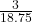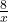## Three books cost $18.75. How much would 8 books cost? Question Three books cost$18.75. How much would 8 books cost?

in progress 0
6 months 2021-07-31T03:49:52+00:00 2 Answers 3 views 0

## Answers ( )

1. Answer: $50 Step-by-step explanation:$18.75 divided by 3 = $6.25$6.25 times 8 = $50 Hope this helps!! 2. Answer:$50

Step-by-step explanation:

Create a proportion where x is the cost of 8 books:=Cross multiply and solve for x:

3x = 150

x = 50

So, 8 books would cost \$50